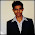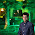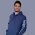## Wednesday, 30 May 2012

### C++ Snippets complied and tested in Turbo C++ (DOS mode).

Q1: Write a simple program to print your name, class and school.
Answer:
```#include <iostream.h>

int main()
{
cout << "My name is Akshay Kumar" << endl;
cout << "Class: XI-A (XYZ School)." << endl;
return 0;
}
```

Q2: Write a program to input a number (say 31) and display it in hexadecimal form (e.g. 0f).
Answer:
```#include <iostream.h>
int main()
{
int x;
cout << Enter an integer:" << endl;
cin >> x;
// hex keyword displays a number in hexadecimal form.
cout << "x=" << hex << x << endl;
cin.get();
return 0;
}
```

Q3: Write a C++ program two swap to integers without taking a temporary variable.

Answer:
```#include <iostream.h>
int main()
{
int a = 10;
int b = 20;
cout << "Value of a (before swap): " << a << endl;
cout << "Value of b (before swap): " << b << endl;
a = a + b;
b = a - b;
a = a - b;
cout << "Value of a (after swap): " << a << endl;
cout << "Value of b (after swap): " << b << endl;
cin.get();
return 0;
}
```

Q4:Write a program to check is a number is multiple of  three or not.

Answer:

```#include <iostream.h>
int main()
{
int x;
cout << Enter an integer:";
cin >> x;
// use % operator to check remainder ```
` if (x % 3)`
`   cout << x << " is NOT a multiple of three." << endl;`
` else`
`   cout << x << " is multiple of three." << endl; `
``` cin.get();
return 0;
}
```

Q5: Write a program to check if a year is a leap year or not.

Answer:
```// program to check if it is a leap year or not
// Note: leap years occur in years exactly divisible by four,
// except that years ending in 00 are leap years
// only if they are divisible by 400.
#include <iostream.h>
#include <conio.h>

int main()
{
int year;
cout << "Enter a year (e.g. 2004): ";
cin >> year;
if ((year % 400 == 0) || ((year %100 != 0) && (year % 4 == 0)))
cout << "The year " << year << " is a leap year" << endl;
else
cout << "The year " << year << " is NOT a leap year" << endl;
getch();
return 0;
}
```

Go to Snippets-2#### 9 comments:

1.Broland has not released any version of Turbo C or C++ that is compatible with Windows 7 or Windows 8. But there are third party Turbo C++ compilers that works fine on Windows 7 and Windows 8.

After searching hrs on google i found Automatic (no need to read and apply complicated tutorial to run C in full screen)
The best of turbo c emulater that is C++ by yogisoft

Download it from http://www.turboc8.com for Free.

Works for all 32 & 64 bit Windows 8,7,xp and vista.

This is All in one solution

Gyzs go for it...
Source(s):
http://www.turboc8.com
http://www.turboc8.blogspot.com

1.C++ by yogisoft is not convienient to use for all... instead i recommend using microsoft visual studio.. which is much faster and more effecient than turbo C++

2.These are not at par with cbse class 11 standard......too low.
-student going fromm xi to xii

3.These are not at par with cbse class 11 standard......too low.
-student going fromm xi to xii

4.The are just not the important programs that appear in class 11 where are the pyramids? Patterns? Series?
~11th student

5.Nice info. Thanks for sharing valuable info about about c++ Faqs. I want to learn c training this tutorial is really helpful. Thanks a lot. Keep sharing on updating tutorials……

6.Yeah..some examples are good BT they are not up to the mark... Try to give some better and challenging examples of which have most probability today appear in exams

7.8.We love to hear your thoughts about this post!